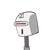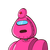# what should subtraction from the sum of 3 2/3 and 2 5/6 to get 5.​

what should subtraction from the sum of 3 2/3 and 2 5/6 to get 5.​

### 2 thoughts on “what should subtraction from the sum of 3 2/3 and 2 5/6 to get 5.​”

1.3 2/3 + 2 5/6 – x = 5

11/3 + 17/6 – x = 5

LCM of 3 & 6 = 6

11/3 X 2/2 = 22/6, 17/6

22+17/6

39/6 – x = 5

Now we will interchange the places of x & 5

39/6 – 5 = x

39/6 – 5/1 = x

LCM of 6 & 1 = 6

39/6, 5/1 X 6/6 = 30/6

39-30/6

9/6 = 1 3/6

If you want to further reduce it, the answer will be 3/2 = 1 1/2

2.let the number be x

(3 2/3 + 2 5/6 ) – x  =  5

(11/3 + 17/6) – x =  5

(44/36 + 51/36) – x = 5

(44+ 51/36) -x = 5

95/36 – x = 5

x = 95× 5/36

x = 475/36